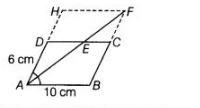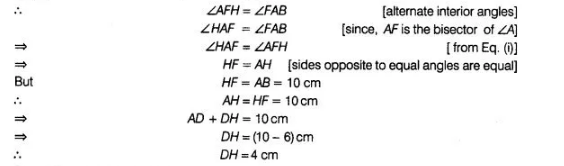# In a parallelogram ABCD, AB = 10 cm`
Question:

In a parallelogram ABCD, AB = 10 cm and AD = 6 cm. The bisector of ∠A meets DC in E. AE and BC produced meet at F. Find the length of CF.

Solution:

Given, a parallelogram ABCD in which AB = 10 cm and AD = 6 cm.

Now, draw a bisector of ∠A meets DC in E and produce it to F and produce BC to meet at F.Also, produce $A D$ to $H$ and join $H F$, so that $A B F H$, is a parallelogram.

Since, $H F \| A B$Since, CFHD is a parallelogram.

Therefore, opposite sides are equal.

$\therefore \quad D H=C F=4 \mathrm{~cm}$